# Producer Surplus

1. Define each of the following terms. Then draw a simple supply and demand graph that illustrates each of these quantities. (You need not put numbers, just show the graph.)
1. Consumer Surplus
2. Producer Surplus
3. Tax Revenue
2. Suppose you have been told that the supply equation for a particular product is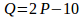and the demand equation is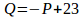. In both cases,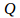is given in thousands. You have also been told that the government is considering a tax of \$1.50 on each item sold.  Answer the following questions:
1. What is the equilibrium point (quantity and price)?
2. What is the consumer surplus, before taxes?
3. What is the producer surplus, before taxes?
4. What is the net social benefit for this market, before taxes?
5. After the tax is imposed, how many fewer items will sell?
6. What is the total revenue generated by the tax?
7. What is the new consumer surplus after taxes?
8. What is the new producer surplus after taxes?
9. What is the deadweight loss for this tax?
10. What is the new net social benefit?

Here are the questions:

1. Consider supply and demand. We have looked at several different aspects of this relationship. Answer the following questions:
1. We have seen that both supply and demand can change at the same time. In particular, if both supply and demand increase, quantity will also increase at equilibrium. Show, however, that we cannot say for sure what will happen to price at the new equilibrium. Draw three graphs. In the first, show that supply and demand can increase and the result can be a price increase. In the second, show that the price might decrease instead. In the third, show that price could also just stay the same. (Hints: Two possible effects to help you show this are differing elasticities and differing sizes of the increases in supply and demand.)
2. What does it mean if I tell you that the price elasticity of demand of apples is 3.2, while the price elasticity of demand of apple juice is 1.5? In your answer, be sure to explain what elasticity is and whether you consider these products to be elastic or inelastic. Finally, suggest reasons why these elasticities might make sense. Are there complements? Substitutes? Explain.
1. Consider supply and demand in the presence of taxation. Suppose you have two different products and you are considering a tax on one or the other of the products. Here are the details for each product:
 Product ASupply Equation: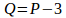Demand Equation: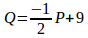(Both are quantities in thousands.) The tax being considered is \$1.50 per item. Product BSupply Equation: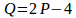Demand Equation: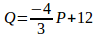(Both are quantities in thousands.) The tax being considered is \$1.75 per item.

1. Which tax will actually raise more tax revenue?
2. Which tax will result in the greatest loss of net benefit (largest deadweight loss)?
3. Based purely on these considerations, which tax would you choose – product A or product B? Explain your decision.
4. What other considerations might affect your decision? (For example, are there potential ethical concerns to watch out for?)
1. What is the prisoners’ dilemma? What do we mean when we say that every market transaction is a solution to the prisoners’ dilemma? How does this relate to the enforcement problem? Explain carefully, using examples liberally to support your explanations.
1. Suppose you have a budget constraint of \$400 dollars for the week and you must decide how to divide the \$400 between exactly two goods. (You must spend it all on the two goods.) The first good costs \$20 per unit and the second good costs \$10 per unit. You have a standard utility function for the items (more is better, but with an eventual diminishing return on each product). Answer the following questions:
1. Which point do you think has a higher utility, 10 of good one and 20 of good two or 12 of good one and 16 of good two? Show that it is possible for a reasonable person to prefer either of these bundles. (Hint: Try different sets of indifference curves.) Do these points satisfy your budget constraint?
2. Using indifference curves, show how you would find the optimal bundle of goods. (You need not use numbers. Just show graphically what a solution would look like.
1. BONUS QUESTION What does it mean to say that decisions should be made at the margins? Explain carefully and thoroughly, using examples to illustrate.
1. ANOTHER BONUS QUESTION Suppose a product sells 500,000 units at a price of \$12 but only 490,000 units at a price of \$16. Answer the following questions:
1. What is the price elasticity of demand for this product, based on these two points? Use the midterm formula to calculate.
2. Is the product elastic or inelastic? Explain.
3. Speculate as to what product this might actually be. Why do you think so? (There is no one right answer, but there are a lot of wrong ones. Choose wisely.)

Do you need help with this assignment or any other? We got you! Place your order and leave the rest to our experts.

Quality Guaranteed